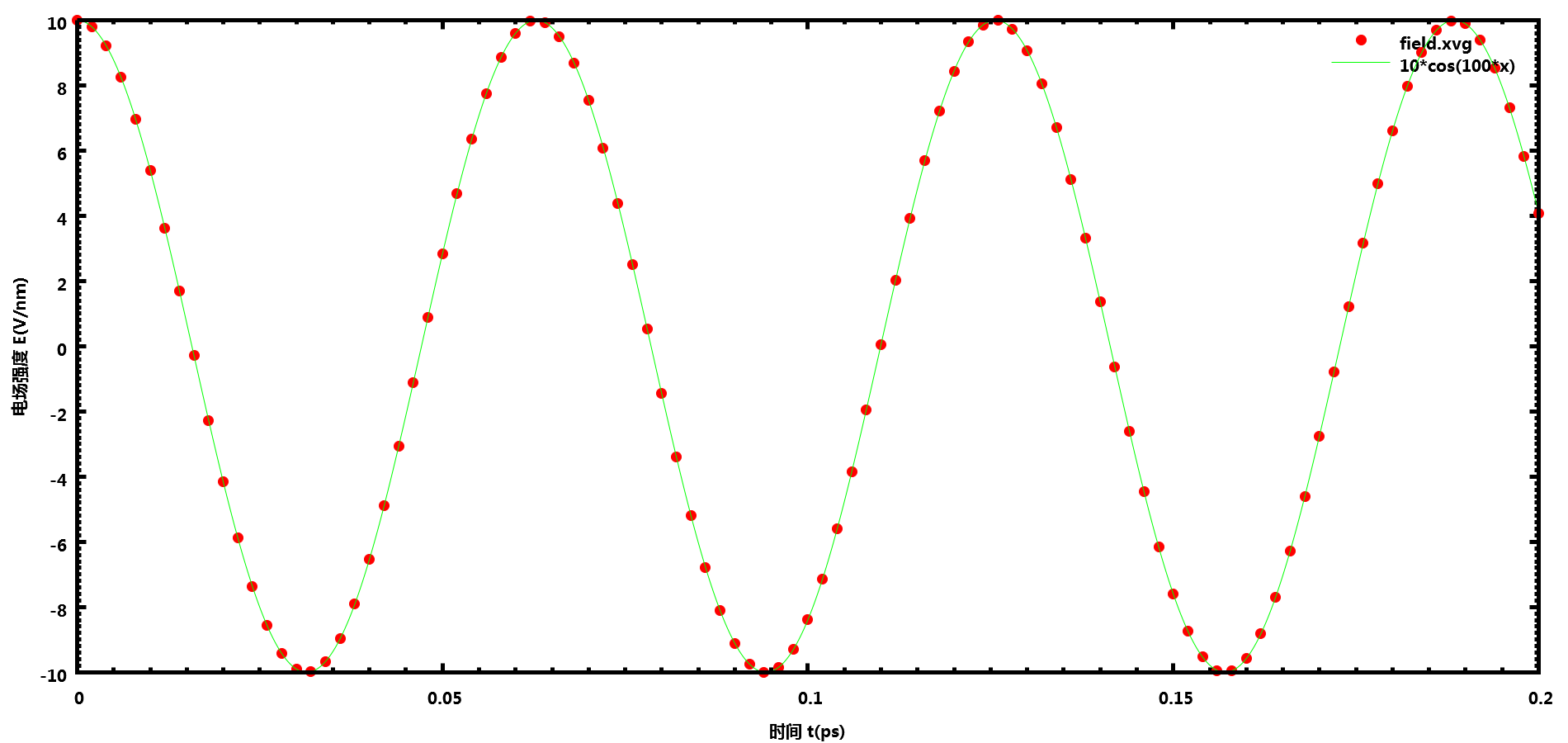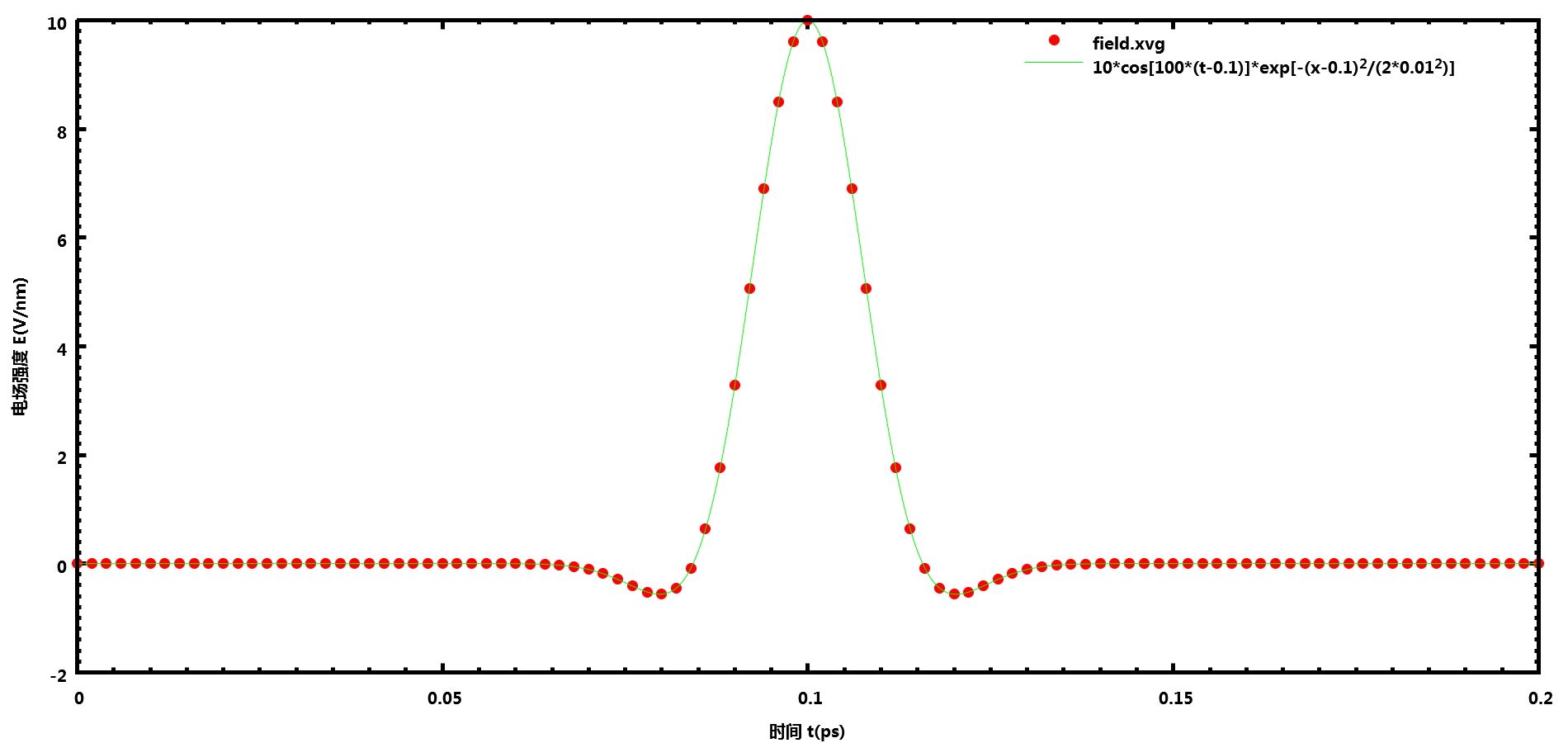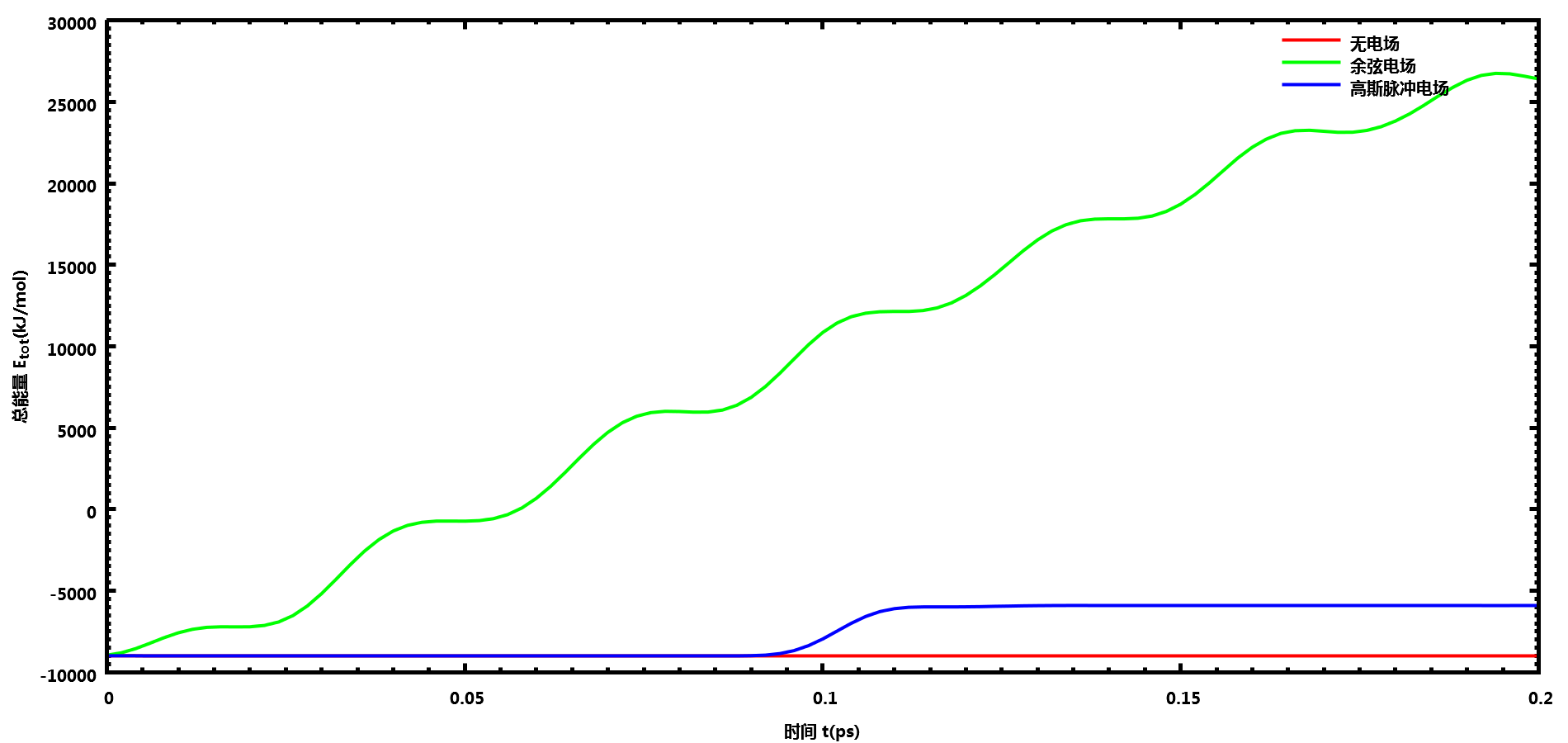# GROMACS电场的使用

• 2016-06-29 11:05:40

GROMACS源代码中有关电场的代码至少可追溯至3.3版本, 但由于低版本手册中没有提及, 所以很多人以为GROMACS是不支持电场的. 在GROMACS 5.x版本的手册中虽然提到了电场的选项, 但仍语焉不详, 且说明与实际代码实现不一致, 导致很多误解. 在这里, 我根据网上查阅到的资料, 以及源代码和实际测试, 对GROMACS的电场选项说明一下. 使用的GROMACS版本为5.1.2.

## 手册7.3.26中电场选项的说明

• E-x; E-y; E-z:

• E-xt; E-yt; E-zt:

$E(t) = E_0 \exp \left[-{(t-t_0)^2 \over 2 \s^2} \right] \cos\left[\w(t-t_0)\right]$

E-x = 1 E0 0

E-xt = omega t0 sigma

E-x等选项一样, E-xt等选项的参数设置格式中, 第一个数字也是余弦项的数目, 后面跟着每个余弦项的频率omega和相位phi, 但源码中没有使用phi, 所以只有设定的omega有意义, phi可以随意给个数字.

## 源代码中的电场部分

GROMACS中电场的实现代码位于.\gromacs-5.1.2\src\gromacs\mdlib\sim_util.cppcalc_f_el函数,

c
 1
2
3
4
5
6
7
8
9
10
11
12
13
14
15
16
17
18
19
20
21
22
23
24
25
26
27
28
29
30
31
32
33
34
35
36
37
38
39
40
41
42
43
44
45
46
47
48
49
50
51
52
53
54
55
56
57
58
59
60
61
62
63
64
65
66
67
/*
* calc_f_el calculates forces due to an electric field.
*
* force is kJ mol^-1 nm^-1 = e * kJ mol^-1 nm^-1 / e
*
* Et[] contains the parameters for the time dependent
* part of the field.
* Ex[] contains the parameters for
* the spatial dependent part of the field.
* The function should return the energy due to the electric field
* (if any) but for now returns 0.
*
* WARNING:
* There can be problems with the virial.
* Since the field is not self-consistent this is unavoidable.
* For neutral molecules the virial is correct within this approximation.
* For neutral systems with many charged molecules the error is small.
* But for systems with a net charge or a few charged molecules
* the error can be significant when the field is high.
* Solution: implement a self-consistent electric field into PME.
*/
static void calc_f_el(FILE *fp, int  start, int homenr,
real charge[], rvec f[],
t_cosines Ex[], t_cosines Et[], double t)
{
rvec Ext;
real t0;
int  i, m;

for (m = 0; (m < DIM); m++)
{
if (Et[m].n > 0)
{
if (Et[m].n == 3)
{
t0     = Et[m].a;
Ext[m] = cos(Et[m].a*(t-t0))*exp(-sqr(t-t0)/(2.0*sqr(Et[m].a)));
}
else
{
Ext[m] = cos(Et[m].a*t);
}
}
else
{
Ext[m] = 1.0;
}
if (Ex[m].n > 0)
{
/* Convert the field strength from V/nm to MD-units */
Ext[m] *= Ex[m].a*FIELDFAC;
for (i = start; (i < start+homenr); i++)
{
f[i][m] += charge[i]*Ext[m];
}
}
else
{
Ext[m] = 0;
}
}
if (fp != NULL)
{
fprintf(fp, "%10g  %10g  %10g  %10g #FIELD\n", t,
Ext[XX]/FIELDFAC, Ext[YY]/FIELDFAC, Ext[ZZ]/FIELDFAC);
}
}


c
 1
2
3
4
5
6
7
8
9
10
11
12
typedef struct {
int   n;    /* Number of terms				*/
real *a;    /* Coeffients (V / nm )                     */
real *phi;  /* Phase angles					*/
} t_cosines;

typedef struct {
real E0;            /* Field strength (V/nm)                        */
real omega;         /* Frequency (1/ps)                             */
real t0;            /* Centre of the Gaussian pulse (ps)            */
real sigma;         /* Width of the Gaussian pulse (FWHM) (ps)      */
} t_efield;


## mdrun时查看电场信息

mdrun运行模拟后, 输出的.log文件中会给出设定的电场信息, 类似于

E-x:
n = 2
a = 1.000000e+001 2.000000e+001
phi = 1.000000e+001 3.000000e+001
E-xt:
n = 3
a = 1.000000e+003 1.000000e-001 1.000000e-002
phi = 0.000000e+000 0.000000e+000 0.000000e+000
E-y:
n = 0
E-yt:
n = 0
E-z:
n = 0
E-zt:
n = 0


mdrun运行时有一个选项-field, 可以指定一个.xvg文件, 里面记录每个时间步的电场大小, 默认输出文件为field.xvg. 利用这个文件你可以查看所加的电场是否正确.

### 余弦电场

.mdp中的设置强度为10 V/nm, 频率为100 ps-1(即100 THz)的余弦电场

E-x              = 1 10 0
E-xt             = 1 100 0


GROMACS输出的数据与设置完全一致### 高斯脉冲电场

.mdp中的设置强度为10 V/nm, 频率为100 ps-1(即100 THz), 脉冲中心0.1 ps, 方差0.01 ps的高斯脉冲电场

E-x              = 1 10 0
E-xt             = 3 100 0 .1 0 .01 0


GROMACS输出的数据与设置完全一致## 使用电场的示例## 评论

• 2017-02-20 14:29:52 hjx 非常不错，学习了。感谢李老师。
◆本文地址: , 转载请注明◆
◆评论问题: https://jerkwin.herokuapp.com/category/3/博客, 欢迎留言◆访问人次(2015年7月 9日起): | 最后更新: 2023-08-17 03:23:12 UTC | 版权所有 © 2008 - 2023 Jerkwin